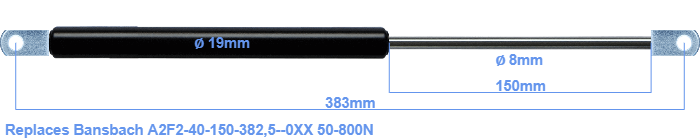# Replacement for Bansbach A2F2-40-150-382,5–0XX 50-800N

\$33.34

Replacement gas spring for the Bansbach A2F2-40-150-382,5--0XX 50-800 Newton. The eye has a thickness of 10mm and a hole diameter of 8.1mm. Brand: HAHN, a Stabilus company.

Please note:
Mounting part rod : The thickness of the eye of this replacement is 10mm instead of 18mm.
 Force Choose an option50 Newton60 Newton80 Newton100 Newton120 Newton140 Newton150 Newton160 Newton180 Newton200 Newton220 Newton240 Newton250 Newton260 Newton280 Newton300 Newton320 Newton340 Newton350 Newton360 Newton380 Newton400 Newton420 Newton440 Newton450 Newton460 Newton480 Newton500 Newton520 Newton540 Newton550 Newton560 Newton580 Newton600 Newton620 Newton640 Newton650 Newton660 Newton680 Newton700 Newton720 Newton740 Newton750 Newton760 Newton780 Newton800 NewtonClearThe diameter of the cylinder of this gas spring is 19 mm. The cross section of the rod is 8 mm. The stroke of the gas spring is 150 millimeter. The total length of this gas strut is 383 mm. Attention: this is the length between the centers of the mounting parts. The distance from thread to thread (without mounting parts) is 345 mm. This gas spring (A2F2-40-150-382,5--0XX) has a force of 50-800 Newton. This gas spring can replace a Bansbach gas spring, but is not an orignal Bansbach one. This gas spring was produced by Stabilus subsidiary HAHN. Nevertheless, the force and dimensions close to equal.